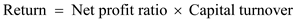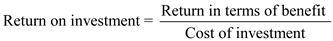## Quiz 19 : Strategic Performance MeasurementLooking for Management Homework Help?# Quiz 19 : Strategic Performance Measurement

Term investment center: The company uses their capital to give the profit in a straight way for company's profitability or can say that it is the treated unit which is used to measure against the use of capital to the cost or profit for the company, known as term investment center or investment division. The measurement of financial performance done by evaluating the return on investment, by using the company can know the probability of performance in a better way the formula is given below:As per the formula, the return is calculated by the multiplication of net profit ratio to capital turnover. The ratio of after-tax profits to the net sales is known as net profit ratio. While the capital turn over can be found by dividing the net annual sales to the average amount of working capital. The financial return or return on investment is used to analyze the money made or lost in an investment at a specific period of time. The financial return may be compared to the alternative investments for rate of return or can say that other investments probability/business units of different types. The investor accepts the cash from the investment like interest division or interest payments of the amount flows and/or consist of the value change of investment are used for the rate of returnee. It can be expressed as percentage of amount invested or in absolute terms.

Return on Investment: Return on investment describes that it is the ratio that lies between net earnings and cost of investment. The high the return on investment the better it is and if the lower the return on investment the worse it is. The return on investment shows the relationship between the return in terms of benefit and the cost of investment. It also measures the performance so that it can evaluate the investment efficiency or it compared the different type of investment efficiency. It can be expressed in a percentage form. The formula to compute the return on investment is as followsBy using the above formula, the return on investment can be easily determined

Rate of investment (ROI): Rate of investment (ROI) is used for assessing the capacity of an investment or do the comparison of the capacity of differently investment's number. High rate of ROI shows that the profit of investment is favorable to cost. ROI is a ratio among the net gain and the cost of investment. Here are the reasons for the issues which have to consider at the time while using the ROI: • Accounting policies' impact which influences the calculation of the income. • The distinction in the impact of the income taxes in units, differential impact of foreign currency exchanges, and the cost allotment's impact when two or more than two units share a service or cost, involved in the other issues for calculating the income. • Investment's calculation: which type of assets to involve • The computation of investment: how to distribute the shared assets' cost